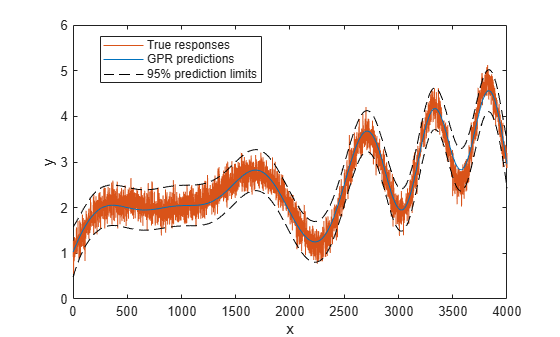# compact

Class: RegressionGP

Create compact Gaussian process regression model

## Syntax

```cgprMdl = compact(gprMdl) ```

## Description

`cgprMdl = compact(gprMdl)` returns a compact version of the trained Gaussian process regression (GPR) model, `gprMdl`.

## Input Arguments

expand all

Gaussian process regression model, specified as a `RegressionGP` object.

## Output Arguments

expand all

Compact Gaussian process regression model, returned as a `CompactRegressionGP` object.

## Examples

expand all

Generate example training data.

```rng(1) % For reproducibility n = 100000; X = linspace(0,1,n)'; X = [X,X.^2]; y = 1 + X*[1;2] + sin(20*X*[1;-2]) + 0.2*randn(n,1);```

Train a GPR model using the subset of regressors (`'sr'`) approximation method and predict using the subset of data (`'sd'`) method. Use 50 points in the active set and sparse greedy matrix approximation (`'sgma'`) method for active set selection. Because the scales of the first and second predictors are different, it is good practice to standardize the data.

```gprMdl = fitrgp(X,y,'KernelFunction','squaredExponential','FitMethod',... 'sr','PredictMethod','sd','Basis','none','ActiveSetSize',50,... 'ActiveSetMethod','sgma','Standardize',1,'KernelParameters',[1;1]);```

`fitrgp` accepts any combination of fitting, prediction, and active set selection methods. In some cases it might not be possible to compute the standard deviations of the predicted responses, hence the prediction intervals. See Tips. And, in some cases, using the exact method might be expensive because of the size of the training data.

Create a compact GPR object.

`cgprMdl = compact(gprMdl);`

Generate the test data.

```n = 4000; Xnew = linspace(0,1,n)'; Xnew = [Xnew,Xnew.^2]; ynew = 1 + Xnew*[1;2] + sin(20*Xnew*[1;-2]) + 0.2*randn(n,1);```

Use the compact object to predict the response in test data and the prediction intervals.

`[ypred,~,yci] = predict(cgprMdl,Xnew);`

Plot the true response, predicted response, and prediction intervals.

```figure; plot(ynew,'r'); hold on; plot(ypred,'b') plot(yci(:,1),'k--'); plot(yci(:,2),'k--'); legend('Data','Pred','Lower 95%','Upper 95%','Location','Best'); xlabel('x'); ylabel('y'); hold off```Compute the mean squared error loss on the test data using the trained GPR model.

`L = loss(cgprMdl,Xnew,ynew)`
```L = 0.0497 ```

## Tips

• The compact object does not contain the training data that `fitrgp` uses in training the GPR model, `gprMdl`.

• You can use the compact object to make predictions (see `predict`) or compute the regression error (see `loss`).

• Computation of standard deviations, `ysd`, and prediction intervals, `yint`, is not supported when `PredictMethod` is `'bcd'`.

• If `gprMdl` is a `CompactRegressionGP` object, you cannot compute standard deviations, `ysd`, or prediction intervals, `yint`, for `PredictMethod` equal to `'sr'` or `'fic'`. To compute `ysd` and `yint` for `PredictMethod` equal to `'sr'` or `'fic'`, use the full regression (`RegressionGP`) object.